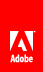# 执行复杂的 3D 转换

Flash Player 10 和更高版本，Adobe AIR 1.5 和更高版本

`var leafMatrix:Matrix3D = this.transform.matrix3D;`

## 创建 Matrix3D 对象

• 使用 Matrix3D() 构造函数方法实例化新的矩阵。 Matrix3D() 构造函数使用包含 16 个数值的 Vector 对象，并将每个值分别放入一个矩阵单元格中。例如：

`var rotateMatrix:Matrix3D = new Matrix3D(1,0,0,1, 0,1,0,1, 0,0,1,1, 0,0,0,1);`
• 设置显示对象的 z 属性的值。然后，从该对象的 transform.matrix3D 属性检索转换矩阵。

• 通过对根显示对象调用 perspectiveProjection.tomatrix3D() 方法，检索用于控制舞台上 3D 对象显示的 Matrix3D 对象。

## 应用多种 3D 转换

```package {
import flash.display.Sprite;
import flash.display.Shape;
import flash.display.Graphics;
import flash.geom.*;

public class Matrix3DTransformsExample extends Sprite
{
private var rect1:Shape;
private var rect2:Shape;

public function Matrix3DTransformsExample():void
{
var pp:PerspectiveProjection = this.transform.perspectiveProjection;
pp.projectionCenter = new Point(275,200);
this.transform.perspectiveProjection = pp;

rect1 = new Shape();
rect1.x = -70;
rect1.y = -40;
rect1.z = 0;
rect1.graphics.beginFill(0xFF8800);
rect1.graphics.drawRect(0,0,50,80);
rect1.graphics.endFill();

rect2 = new Shape();
rect2.x = 20;
rect2.y = -40;
rect2.z = 0;
rect2.graphics.beginFill(0xFF0088);
rect2.graphics.drawRect(0,0,50,80);
rect2.graphics.endFill();

doTransforms();
}

private function doTransforms():void
{
rect1.rotationX = 15;
rect1.scaleX = 1.2;
rect1.x += 100;
rect1.y += 50;
rect1.rotationZ = 10;

var matrix:Matrix3D = rect2.transform.matrix3D;
matrix.appendRotation(15, Vector3D.X_AXIS);
matrix.appendScale(1.2, 1, 1);
matrix.appendTranslation(100, 50, 0);
matrix.appendRotation(10, Vector3D.Z_AXIS);
rect2.transform.matrix3D = matrix;
}
}
}```

doTransforms() 方法中，第一个代码块使用 DisplayObject 属性更改矩形形状的旋转、缩放和位置。第二个代码块使用 Matrix3D 类的方法执行相同的转换。

## 使用 Matrix3D 对象重新排序显示

1. 使用 Transform 对象的 getRelativeMatrix3D() 方法获取子级 3D 显示对象的相对 z 轴 值。

2. 使用 removeChild() 方法从显示列表中删除对象。

3. 根据显示对象的相对 z 轴值对显示对象进行排序。

```public var faces:Array; . . .

public function ReorderChildren()
{
for(var ind:uint = 0; ind < 6; ind++)
{
faces[ind].z = faces[ind].child.transform.getRelativeMatrix3D(root).position.z;
this.removeChild(faces[ind].child);
}
faces.sortOn("z", Array.NUMERIC | Array.DESCENDING);
for (ind = 0; ind < 6; ind++)
{